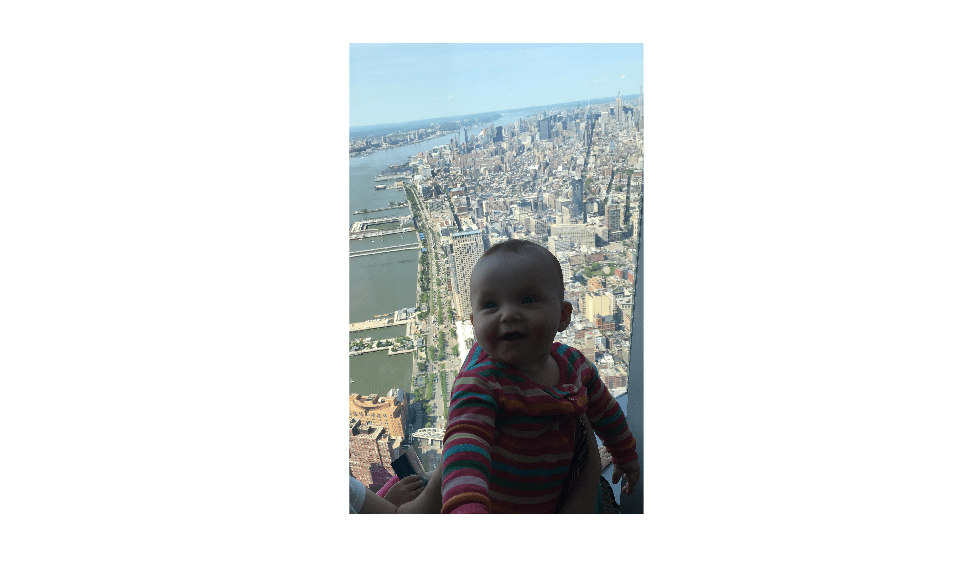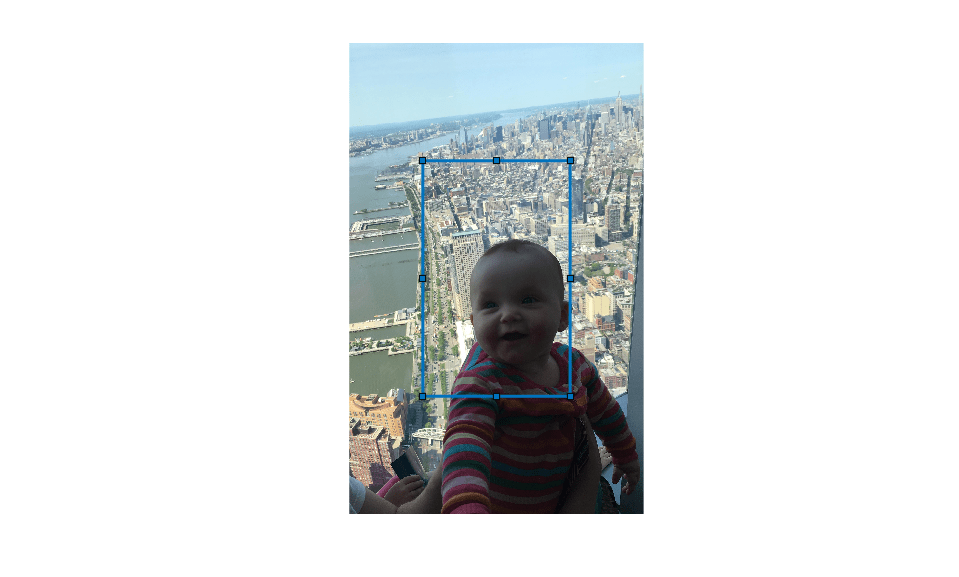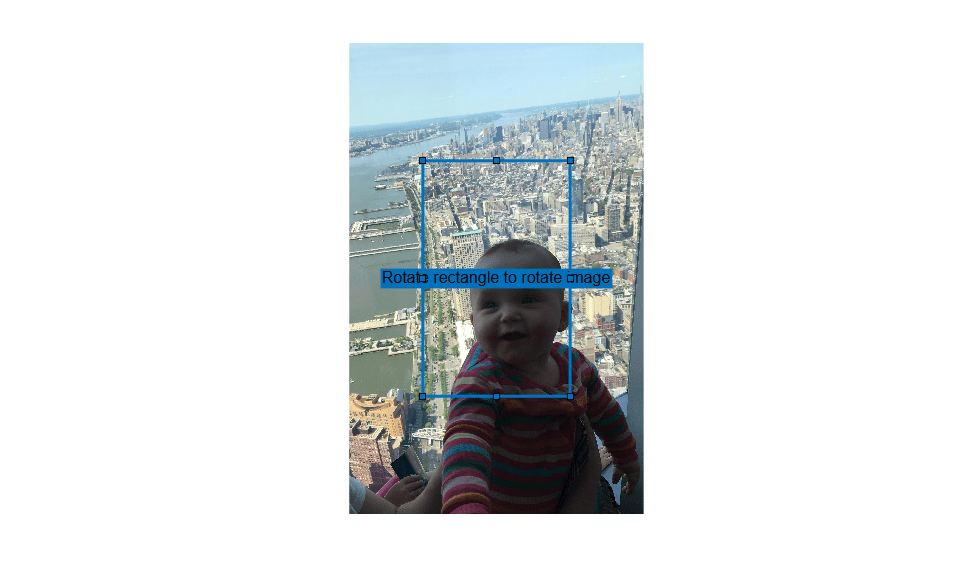Main Content

# Rotate Image Interactively Using Rectangle ROI

This example shows how to rotate an image by using a Rectangle ROI with a callback function that calls `imrotate` when you move the ROI.

Image rotation is a common preprocessing step. In this example, an image needs to be rotated by an unknown amount to align the horizon with the x-axis. You can use the `imrotate` function to rotate the image, but you need prior knowledge of the rotation angle. By using an interactive rotatable ROI, you can rotate the image in real time to match the rotation of the ROI.

### Create the Rotatable Rectangle ROI

Display an image in an Axes.

```im = imread('baby.jpg'); hIm = imshow(im); ```Get the size of the image.

```sz = size(im); ```

Determine the position and size of the Rectangle ROI as a 4-element vector of the form [x y w h]. The ROI will be drawn at the center of the image and have half of the image width and height.

```pos = [(sz(2)/4) + 0.5, (sz(1)/4) + 0.5, sz(2)/2, sz(1)/2]; ```

Create a rotatable Rectangle ROI at the specified position and set the Rotatable property to `true`. You can then rotate the rectangle by clicking and dragging near the corners. As the ROI moves, it broadcasts an event MovingROI. By adding a listener for that event and a callback function that executes when the event occurs, you can rotate the image in response to movements of the ROI.

```h = drawrectangle('Rotatable',true,... 'DrawingArea','unlimited',... 'Position',pos,... 'FaceAlpha',0); ```Place a prompt in the label.

```h.Label = 'Rotate rectangle to rotate image'; ```Add a listener that listens for any movement of the ROI.

```addlistener(h,'MovingROI',@(src,evt) rotateImage(src,evt,hIm,im)); ```

### Call `imrotate` in Callback Function

Define a callback function that executes as the Rectangle ROI moves. This function retrieves the current rotation angle of the ROI, calls `imrotate` on the image with that rotation angle, and updates the display. The function also updates the label to display the current rotation angle.

```function rotateImage(src,evt,hIm,im) % Only rotate the image when the ROI is rotated. Determine if the % RotationAngle has changed if evt.PreviousRotationAngle ~= evt.CurrentRotationAngle % Update the label to display current rotation src.Label = [num2str(evt.CurrentRotationAngle,'%30.1f') ' degrees']; % Rotate the image and update the display im = imrotate(im,evt.CurrentRotationAngle,'nearest','crop'); hIm.CData = im; end end ```Download ebook